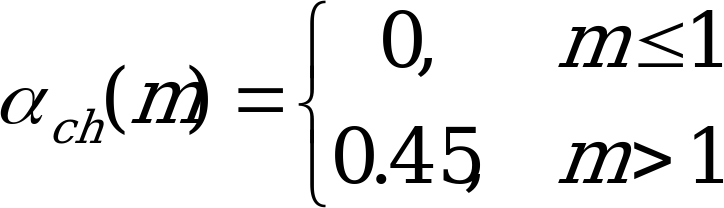## 4.3.2 Channel Energy Estimator

06.943GPPTSVoice Activity Detector (VAD) for Adaptive Multi Rate (AMR) speech traffic channels

Calculate the channel energy estimate Ech(m) for the current subframe, m, as:

(4.4)

where Emin is the minimum allowable channel energy, ch(m) is the channel energy smoothing factor (defined below), Nc is the number of combined channels, and fL(i) and fH(i) are the i-th elements of the respective low and high channel combining tables.

The channel energy smoothing factor, ch(m), is defined as:(4.5)

So, this means that ch(m) assumes a value of zero for the first frame (m = 1) and a value of 0.45 for all subsequent frames. This allows the channel energy estimate to be initialized to the unfiltered channel energy of the first frame.